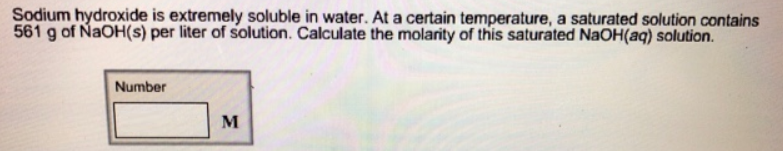# Problem: Sodium hydroxide is extremely soluble in water. At a certain temperature, a saturated solution contains 561 g of NaOH(s) per liter of solution. Calculate the molarity of this saturated NaOH(aq) solution.

###### FREE Expert Solution
95% (257 ratings)###### Problem Details

Sodium hydroxide is extremely soluble in water. At a certain temperature, a saturated solution contains 561 g of NaOH(s) per liter of solution. Calculate the molarity of this saturated NaOH(aq) solution.Frequently Asked Questions

What scientific concept do you need to know in order to solve this problem?

Our tutors have indicated that to solve this problem you will need to apply the Molarity concept. You can view video lessons to learn Molarity. Or if you need more Molarity practice, you can also practice Molarity practice problems.

What professor is this problem relevant for?

Based on our data, we think this problem is relevant for Professor Hascall's class at NAU.